Home
Hostname: page-component-59b7f5684b-9m8n8 Total loading time: 0.875 Render date: 2022-09-24T17:51:15.288Z Has data issue: true Feature Flags: { "shouldUseShareProductTool": true, "shouldUseHypothesis": true, "isUnsiloEnabled": true, "useRatesEcommerce": false, "displayNetworkTab": true, "displayNetworkMapGraph": false, "useSa": true } hasContentIssue true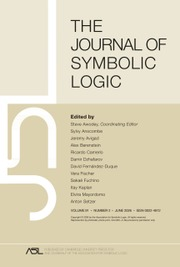The Journal of Symbolic Logic

# MARGINALIA ON A THEOREM OF WOODIN

Published online by Cambridge University Press:  21 March 2017

## Abstract

Let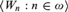$\left\langle {{W_n}:n \in \omega } \right\rangle$ be a canonical enumeration of recursively enumerable sets, and suppose T is a recursively enumerable extension of PA (Peano Arithmetic) in the same language. Woodin (2011) showed that there exists an index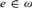$e \in \omega$ (that depends on T) with the property that if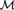${\cal M}$ is a countable model of T and for some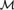${\cal M}$-finite set s,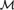${\cal M}$ satisfies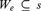${W_e} \subseteq s$, then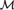${\cal M}$ has an end extension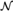${\cal N}$ that satisfies T + We = s.

Here we generalize Woodin’s theorem to all recursively enumerable extensions T of the fragment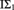${{\rm{I}\rm{\Sigma }}_1}$ of PA, and remove the countability restriction on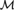${\cal M}$ when T extends PA. We also derive model-theoretic consequences of a classic fixed-point construction of Kripke (1962) and compare them with Woodin’s theorem.

## Keywords

Type
Articles
Information
The Journal of Symbolic Logic , March 2017 , pp. 359 - 374
Copyright © The Association for Symbolic Logic 2017

## Access options

Get access to the full version of this content by using one of the access options below. (Log in options will check for institutional or personal access. Content may require purchase if you do not have access.)

## References

#### REFERENCES

Avigad, J., Formalizing forcing arguments in subsystems of second-order arithmetic . Annals of Pure and Applied Logic, vol. 82 (1996), pp. 165191.CrossRefGoogle Scholar
Beklemishev, L. and Visser, A., On the limit existence principles in elementary arithmetic and${\rm{\Sigma }}_n^0$ -consequences of theories . Annals of Pure and Applied Logic, vol. 136 (2005), pp. 5674.CrossRefGoogle Scholar
Blanck, R., Two consequences of Kripke’s lemma , Idées Fixes (Kaså, Martin, editor), University of Gothenburg Publications, 2014, pp. 4553.Google Scholar
Boolos, G., The Logic of Provability, Cambridge University Press, Cambridge, 1979.Google Scholar
Cornaros, C., Versions of Friedman’s theorem for fragments of PA, http://www.softlab.ntua.gr/∼nickie/tmp/pls5/cornaros.pdf.
D’Aquino, P., A sharpened version of McAloon’s theorem on initial segments of0 . Annals of Pure and Applied Logic, vol. 61 (1993), pp. 4962.CrossRefGoogle Scholar
Dimitracopoulos, C. and Paris, J., A note on a theorem of H. Friedman . Zeitschrift für mathematische Logik und Grundlagen der Mathematik, vol. 34 (1988), pp. 1317.CrossRefGoogle Scholar
Dimitracopoulos, C. and Paschalis, V., End extensions of models of weak arithmetic theories . Notre Dame Journal of Formal Logic, to appear.
Enayat, A. and Wong, T., Model theory of${\rm{WKL}}_0^{^{\rm{*}}}$ . Annals of Pure and Applied Logic, to appear.
Guaspari, D., Partially conservative extensions of arithmetic . Transaction of the American Mathematical Society, vol. 254 (1979), pp. 4768.CrossRefGoogle Scholar
Hájek, P., Interpretability and fragments of arithmetic . Arithmetic, Proof Theory, and Computational Complexity, Oxford University Press, Oxford, 1993, pp. 185196.Google Scholar
Hájek, P. and Pudlák, P., Metamathematics of First-Order Arithmetic, Springer-Verlag, Berlin, 1993.CrossRefGoogle Scholar
Japaridze, G. and de Jongh, D., The logic of provability , Handbook of Proof Theory, North-Holland, Amsterdam, 1998, pp. 475546.CrossRefGoogle Scholar
Kaye, R., Models of Peano Arithmetic, Oxford University Press, Oxford, 1991.Google Scholar
Kossak, R. and Schmerl, J., The Structure of Models of Peano Arithmetic, Oxford University Press, Oxford, 2006.CrossRefGoogle Scholar
Kripke, S., “Flexible” predicates of formal number theory . Proceedings of the American Mathematical Society, vol. 13 (1962), pp. 647650.Google Scholar
Lindström, P., Aspects of Incompleteness, Lecture Notes in Logic, vol. 10, ASL Publications, 1997.CrossRefGoogle Scholar
McAloon, K., On the complexity of models of arithmetic, this Journal, vol. 47 (1982), pp. 403415.
Ressayre, J.-P., Nonstandard universes with strong embeddings, and their finite approximations , Logic and Combinatorics, Contemporary Mathematics, vol. 65, American Mathematical Society, Providence, RI, 1987, pp. 333358.CrossRefGoogle Scholar
Rogers, H., Theory of Recursive Functions and Effective Computability, McGraw-Hill, Cambridge, MA, 1967.Google Scholar
Shen, A., Are random axioms useful? Math. ArXiv, http://arxiv.org/pdf/1109.5526.pdf.
Simpson, S., Subsystems of Second Order Arithmetic, Springer-Verlag, Berlin, 1999.CrossRefGoogle Scholar
Smoryński, C., Modal Logic and Self-reference, Springer-Verlag, Berlin, 1985.CrossRefGoogle Scholar
Verbrugge, R. and Visser, A., A small reflection principle for bounded arithmetic, this Journal, vol. 59 (1994), pp. 785812.
Woodin, W. H., A potential subtlety concerning the distinction between determinism and nondeterminism , Infinity, New Research Frontiers (Heller, M. and Woodin, W. H., editors), Cambridge University Press, Cambridge, 2011, pp. 119129.CrossRefGoogle Scholar
1
Cited by

# Save article to Kindle

To save this article to your Kindle, first ensure coreplatform@cambridge.org is added to your Approved Personal Document E-mail List under your Personal Document Settings on the Manage Your Content and Devices page of your Amazon account. Then enter the ‘name’ part of your Kindle email address below. Find out more about saving to your Kindle.

Note you can select to save to either the @free.kindle.com or @kindle.com variations. ‘@free.kindle.com’ emails are free but can only be saved to your device when it is connected to wi-fi. ‘@kindle.com’ emails can be delivered even when you are not connected to wi-fi, but note that service fees apply.

Find out more about the Kindle Personal Document Service.

MARGINALIA ON A THEOREM OF WOODIN
Available formats
×

# Save article to Dropbox

To save this article to your Dropbox account, please select one or more formats and confirm that you agree to abide by our usage policies. If this is the first time you used this feature, you will be asked to authorise Cambridge Core to connect with your Dropbox account. Find out more about saving content to Dropbox.

MARGINALIA ON A THEOREM OF WOODIN
Available formats
×

# Save article to Google Drive

To save this article to your Google Drive account, please select one or more formats and confirm that you agree to abide by our usage policies. If this is the first time you used this feature, you will be asked to authorise Cambridge Core to connect with your Google Drive account. Find out more about saving content to Google Drive.

MARGINALIA ON A THEOREM OF WOODIN
Available formats
×
×